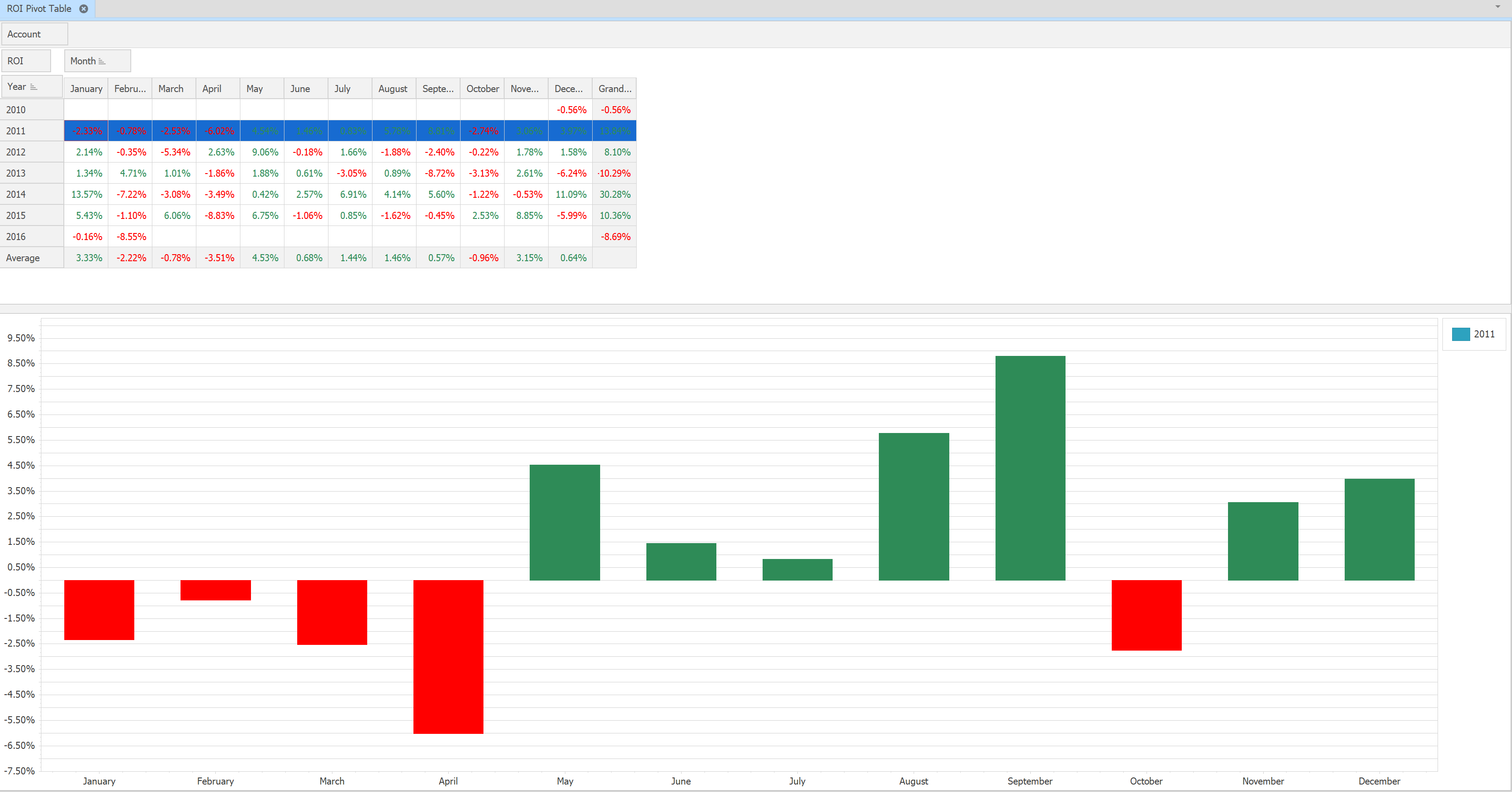﻿ Reports > Equity > ROI Pivot Table

ROI Pivot Table

ROI Pivot Table is a special grid where you can see monthly return on investment values based on the total equity list.

The monthly values are calculated with the following formula:

ROI value = (Ending equity - Starting Equity - Offset) / Starting Equity where

Ending Equity - equity(net asset) value at the end of the month

Starting Equity - equity value at the end of the previous month

Offset - sum of deposits and withdrawals during the month